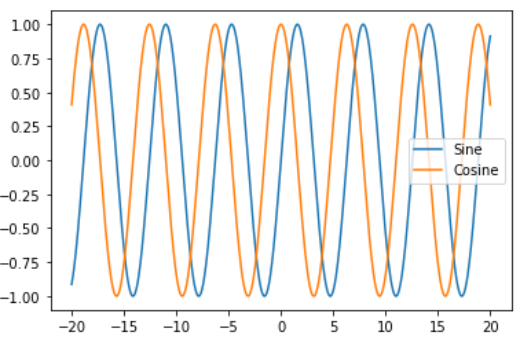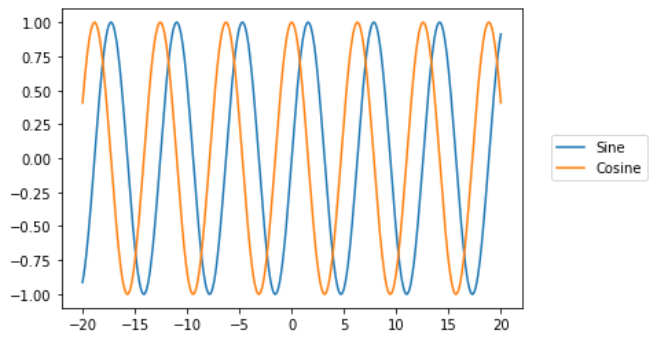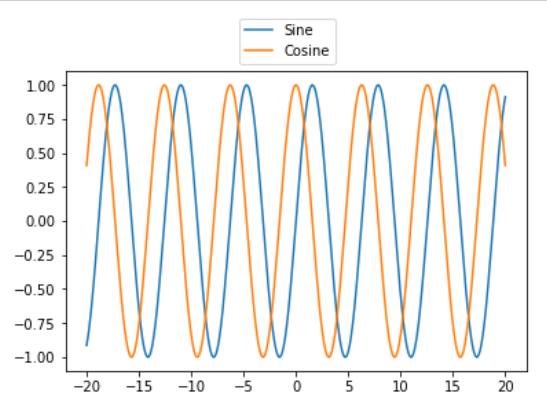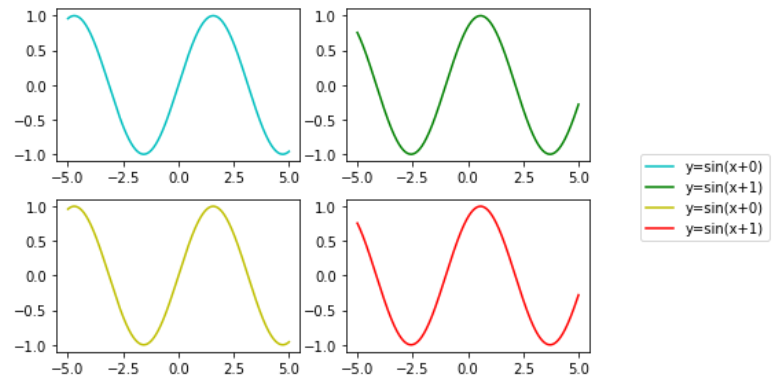# How to Place Legend Outside of the Plot in Matplotlib?

• Last Updated : 03 Jan, 2021

In this article, we will see how to place legend outside the Plot in Matplotlib? Let’s discuss some concepts :

• Matplotlib : Matplotlib is an amazing visualization library in Python for 2D plots of arrays. Matplotlib is a multi-platform data visualization library built on NumPy arrays and designed to work with the broader SciPy stack. It was introduced by John Hunter in the year 2002.
• Legend : A legend is an area describing the elements of the graph. In the matplotlib library, there’s a function called legend() which is used to Place a legend on the axes. The attribute Loc in legend() is used to specify the location of the legend. Default value of loc is loc=”best” (upper left).

Here, first we will see why placing of legend outside is required.

Attention geek! Strengthen your foundations with the Python Programming Foundation Course and learn the basics.

To begin with, your interview preparations Enhance your Data Structures concepts with the Python DS Course. And to begin with your Machine Learning Journey, join the Machine Learning - Basic Level Course

## Python3

 `# importing packages``import` `numpy as np``import` `matplotlib.pyplot as plt`` ` `# create data``x``=``np.linspace(``-``20``, ``20``, ``1000``)`` ` `# plot the graphs``plt.plot(x,np.sin(x))``plt.plot(x,np.cos(x))`` ` `# add legends``plt.legend([``"Sine"``,``"Cosine"``])`` ` `plt.show()`

Output:Legends overlap the graph (incomplete information)

As, we can see that the above figure legends are overlapped on graph i.e; incomplete information. To solve this problem we need to place the legend outside the plot.

### Steps Needed

1. Import Libraries
3. Make plots
4. Add legend outside the plot.

Example 1: (Right Side)

## Python3

 `# importing packages``import` `numpy as np``import` `matplotlib.pyplot as plt`` ` `# create data``x``=``np.linspace(``-``20``, ``20``, ``1000``)`` ` `# plot the graphs``plt.plot(x,np.sin(x))``plt.plot(x,np.cos(x))`` ` `# add legends and set its box position``plt.legend([``"Sine"``,``"Cosine"``],``           ``bbox_to_anchor ``=` `(``1.05``, ``0.6``))`` ` `plt.show()`

Output:Example 2: ( At top)

## Python3

 `# importing packages``import` `numpy as np``import` `matplotlib.pyplot as plt`` ` `# create data``x``=``np.linspace(``-``20``, ``20``, ``1000``)`` ` `# plot the graphs``plt.plot(x, np.sin(x))``plt.plot(x, np.cos(x))`` ` `# add legends and set its box position``plt.legend([``"Sine"``, ``"Cosine"``],``           ``bbox_to_anchor``=``(``0.6``, ``1.2``))`` ` `plt.show()`

Output :Example 3: ( With subplots)

## Python3

 `# importing packages``import` `numpy as np``import` `matplotlib.pyplot as plt`` ` `# create data``x ``=` `np.linspace(``-``5``, ``5``, ``1000``)``colors``=``[[``'c'``,``'g'``], [``'y'``,``'r'``]]`` ` `# make subplot and plots the grpahs``fig, ax ``=` `plt.subplots(``2``, ``2``)``for` `i ``in` `range``(``2``):``    ``ax[``0``][i].plot(x, np.sin(x``+``i),``                  ``color ``=` `colors[``0``][i],``                  ``label ``=` `"y=sin(x+{})"``.``format``(i))``     ` `    ``ax[``1``][i].plot(x, np.sin(x``+``i), ``                  ``color ``=` `colors[``1``][i],``                  ``label ``=` `"y=sin(x+{})"``.``format``(i))``     ` `# set legend position``fig.legend(bbox_to_anchor``=``(``1.3``, ``0.6``))`` ` `# set spacing to subplots``fig.tight_layout()  ``plt.show()`

Output :My Personal Notes arrow_drop_up